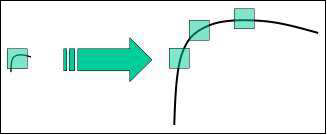# OpenCV Python - Feature Detection

In the context of image processing, features are mathematical representations of key areas in an image. They are the vector representations of the visual content from an image.

Features make it possible to perform mathematical operations on them. Various computer vision applications include object detection, motion estimation, segmentation, image alignment etc.

Prominent features in any image include edges, corners or parts of an image. OpenCV supports Haris corner detection and Shi-Tomasi corner detection algorithms. OpenCV library also provides functionality to implement SIFT (Scale-Invariant Feature Transform), SURF(Speeded-Up Robust Features) and FAST algorithm for corner detection.

Harris and Shi-Tomasi algorithms are rotation-invariant. Even if the image is rotated, we can find the same corners. But when an image is scaled up, a corner may not be a corner if the image. The figure given below depicts the same.D.Lowe's new algorithm, Scale Invariant Feature Transform (SIFT) extracts the key points and computes its descriptors.

This is achieved by following steps −

• Scale-space Extrema Detection.
• Keypoint Localization.
• Orientation Assignment.
• Keypoint Descriptor.
• Keypoint Matching.

As far as implementation of SIFT in OpenCV is concerned, it starts from loading an image and converting it into grayscale. The cv.SHIFT_create() function creates a SIFT object.

## Example

Calling its detect() method obtains key points which are drawn on top of the original image. Following code implements this procedure

import numpy as np
import cv2 as cv
gray= cv.cvtColor(img,cv.COLOR_BGR2GRAY)
sift = cv.SIFT_create()
kp = sift.detect(gray,None)
img=cv.drawKeypoints(gray,kp,img)
cv.imwrite('keypoints.jpg',img)


## Output

The original image and the one with keypoints drawn are shown below −

This is an original image.An image given below is the one with keypoints